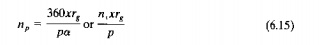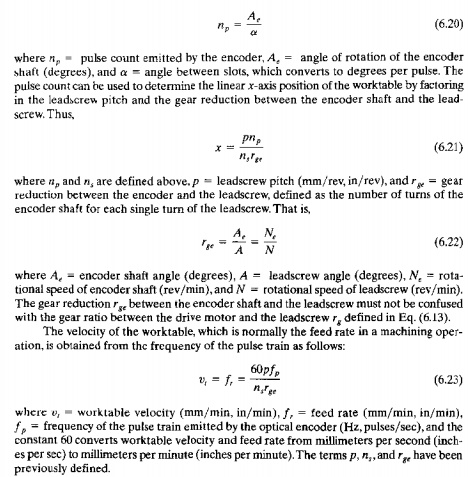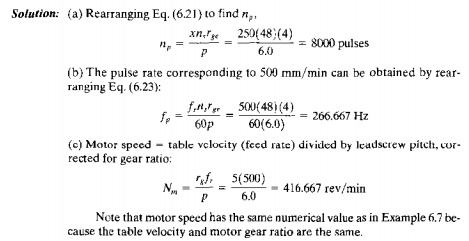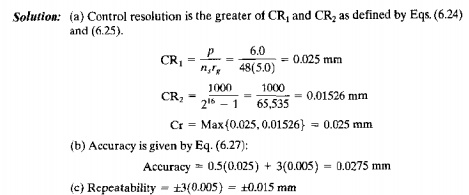Home | | Automation Production System and Computer Integrated Manuacturing | Engineering Analysis of Numerical Control(NC) Positioning Systems

# Engineering Analysis of Numerical Control(NC) Positioning Systems

Engineering Analysis of NC Positioning Systems: a. Open Loop Positioning Systems b. Closed Loop Positioning Systems c. Precision in NC Positioning

ENGINEERING  ANALYSIS  OF NC POSITIONING  SYSTEMS

The NCpositioning system converts the coordinate axis values in the NC part program into relative positions of the tool and workpart during processing. Let us consider the simple positioning system shown in Figure 6.26. The system consists of a cutting tool and a worktable on which a workpart is fixtured. The table is designed to move the part relative to the tool. The worktable moves linearly b)' means of a rotating leadscrew, which is driven by a stepping motor or servomotor. For simplicity, we show only one axis in our sketch. To provide .~.y capability: the system shown would be piggybacked on top of a second axis per, pendicular to the first. The leadscrew bas a certain pitchp (in/thread, mm/thread). Thus, the table mows a distance equal to the pitch for each revolution. The velocity of the worktable, which corresponds to the feed rate in a machining operation, is determined by the rotational speed 01 the leadscrew,There  are two types  of positioning systems  used  in NC systems:  (a) open  loop and (b) closed loop, as shown in Figure 6.27. An open-loop system operates without verifying that the actual position achieved in the move is the same as the desired position. A closed loop control system uses feedback measurements to confirm that the final position of the worktable is the location specified in the program. Open loop systems cost less than closedloop systems and are appropriate when the force resisting the actuating motion is minimal. Closed loop systems are normally specified for machines that perform continuous path operations such as milling or turning, in which there are significant forces resisting the forward motion of the Cuting tool.Open Loop  Postioning Systems

An openloop positioning system typically uses a stepping motor to rotate the leadscrew A stepping motor is driven by a series of electrical pulses, which are generated by the MCU in an NC system. Each pulse causes the motor to rotate a fraction of one revolution. called the step angle. The possible step angles must be consistent with the following relationship:where ct = step angle (degrees), and n, = the number of step angles for the motor, which must be an integer. The angle through which the motor shaft rotates is given bywhere Am = angle of motor shaft rotation (degrees), np == number of pulses received by the motor. and ct = step angle (degrees/pulse). The motor shaft is generally connected to the leadscrew through a gear box, which reduces the angular rotation of the leadscrew. The angle of the leadserew rotation must take the gear ratio into account as follows'where A == angle of leadscrew rotation (degrees), and r g = gear ratio, defined as the number of turns of the molor for each single turn of the leadserew. That is,where x == xaxis position relative to the starting position (mm, inch), p = pitch of the leadscrew (nun/rev, in/rev).and Aj360 = number of Ieadscrew revolutions, The number of pulses required to achieve a specified .rposition increment in a pointtopoint system can be found by combining the two preceding equations as follows:where the second expression on the righthand side is obtained by substituting n, for 360/ a, which is obtained by rearranging Eq. (6.10).

Control pulses are transmitted from the pulse generator at a certain frequency, which drives the worktable at a corresponding velocity or feed rate in the direction of the leadscrew axis. The rotational speed of the leadscrew depends on the frequency of the pulse train as follows:EXAMPLE 6.7    NC Open-Loop       Positioning

The worktable of a positioning system is driven by a leadserew whose pitch == 6.0 mm. The leadscrew is connected to the output shaft of a stepping motor through a gearbox whose ratio ts S: 1 (5 turns of the motor to one turn of the leadscrew). The stepping motor has 48 step angles. The table must move a distance of 250 mm from its present position at a linear velocity '" 500 mm/min Determine (a) how many pulses are required to move the table the specified distance and (b) the required motor speed and pulse rate to aehieve the desired table velocity,

Solution: (a) Rearranging Eq. (6.14) to find the teadscrew rotation angle A corresponding to a distance x = 250 mm,Closed Loop   Positioning   Systems

A closed-loop NC system, illustrated in Figure 6.27(h). uses servomotors and feedback measurements to ensure that the worktable is moved to the desired position. A common feedback sensor used for NC (and also for industrial robots) is the optical encoder, shown in Figure 6.28. An optical encoder consists of a light source and a photodetector on either side of a disk. The disk contains slots uniformly spaced around the outside of its face. These slots allow the light source to shine through and energize the photodetector. The disk is connected, either directly or through a gear box, to a rotating shaft whose angular position and velocity are to be measured. As the shaft rotates, the slots cause the light source to be seen by the photocell as a series of flashes. The flashes are converted into an equal number of electrical pulses. By counting the pulses and computing the frequency of the pulse train, worktable position and velocity can be determined.

The equations that define the operation of a closedloop NC positioning system are similar to those for an openloop system. In the basic optical encoder, the angle between slots in the disk must satisfy the following requirement:where np = pulse count emitted by the encoder, Ae = angle of rotation of the encoder shan (degrees), and a = angle between slots, which converts to degrees per pulse. The pulse count can he used to determine the linear xaxis position of the worktable by factoring in the leadscrew pitch and the gear reduction between the encoder shaft and the leadscrew. Thus,where np and n, are defined above,p = leadscrew pitch (mm/rev, in/rev), and r ge = gear reduction between the encoder and the Ieadscrew, defined as the number of turns of the encoder shaft for each single turn of the leadscrew, That is,The pulse train generated by the encoder is compared with the coordinate position and feed rate specified in the part program, and the difference is used by the MCV to drive a servomotor, which in tum drives the worktable. A digitaltoanalog converter (Section 5.4) converts the digital signals used by the MeV into a continuous analog current that powers the drive motor. Closedloop NC systems of the type described here are appropriate when a reactionary force resists the movement of the table. Metal cutting machine tools that perform continuous path cutting operations, such as milling and turning, fall into this category.

EXAMPLE         6,8      NC ClosedLoop   PositioninA

An NC worktable operates by closedloop positioning. The system consists of a servomotor, leadscrew, and optical encoder. The leadscrew has a pitch = 6.0 rom

and is coupled to the motor shaft with a gear ratio of 5: I (5 turns of the drive motor for each turn of the leadscrcw). The optical encoder generates 48 pulses/rev of its output shaft. The encoder output shaft is coupled to the Ieadscrew with a 4: 1 reduction (4 turns of the encoder shaft for each turn of the leadscrew). The table has been programmed to move a distance of 250 mm at a feed rate = 500 mm/min. Determine (a] how many pulses should be received by the control system to verify that the table has moved exactly 250 mm, (b) the pulse rate of the encoder, and (c) the drive motor speed that correspond to the specified feed rate.Precision  in NC Positioning

For accurate machining or other processing performed by an NC system, the positioning system must possess a high degree of precision. Three measures of precision can be defined for an NC positioning system: (I) control resolution. (2) accuracy, and (3) repeatability.

These terms are most readily explained by considering a single axis of the positioning system, as depicted in Figure 6.29. Control resolution refers to the control system's ability todivide the total range of the axis movement into closely spaced points that can be distinguished bythe Men Control resolution is defined as the distance separating two adjacent addressable points in the axis movement. Addressable points are locations along the axis to which the worktable can be specifically directed to go. It is desirable for control resolution to be as small as possible. This depends on limitations imposed by: (1) the electromechanical components of the positioning system and/or (2) the number of bits used by the controller to define the axis coordinate location.

A number of electromechanical factors affect control resolution, including: leadscrew pitch, gear ratio in the drive system, and the step angle in a stepping motor for an openloop system or the angle between slots in an encoder disk for a closedloop system. For an openloop positioning system driven by a stepper motor, these factors can be combined into an expression that defines control resolution as follows:A desirable criterion is for CR2 <= CR1, meaning that the electromechanical system is the bmiting factor that determines control resolution. The bit storage capacity of a modem computer controller is sufficient to satisfy this criterion except in unusual situations. Resolutions of 0.0025 mm (0,0001 in) are within the current state of NC technology.

The capability of a positioning system to move the worktable to the exact location defined by a given addressable point is limited by mechanical errors that are due to various imperfections in the mechanical system. These imperfections include play between the leadscrew and the worktable, backlash in the gears, and deflection of machine components.

We assume that the mechanical errors form an unbiased normal statistical distribution about tle control point whose mean IJ. = D. We further assume that the standard deviation IT of the distribution is constant over the range of the axis under consideration. Given these assumptions, then nearly all of the mechanical errors (99.74%) are contained within ±3r:r of the control point. This is pictured in figure 1i.29for a portion of the axis range that in

cludes  two control  points.

Let us now make use of these definitions of control resolution and mechanical error distribution to define accuracy and repeatability of a positioning system. Accuracy is defined under 'Worst case conditions in which the desired target point lies in the middle between two adjacent addressable points. Since the table can only be moved to one or the other of the addressable points, there will be an error in the final position of the worktable. This is the maximum possible positioning error, because if the target were closer to either one of the addressable points, then the table would be moved to the closer control point and the error would be smaller. It is appropriate to define accuracy under this worst case scenario. The accuracy of any given axis of a positioning system is the maximum possible error that can occur between the desired target point and the actual position taken by the system'. in equation form,where CR = control resolution (mm,in), and o = standard deviation of the error distribution. Accuracies in machine tools are generally expressed for a certain range of table travel.tor example.Lo.Ot mm for 250mm (±OJlOO4 in. for 10 in) of table travel

Repeatability refers to the capability of the positioning system to return to a given addressable point that has been previously programmed. This capability can be measured in terms of the location errors encountered when the system attempts to position itself at the addressable point. Location errors are a manifestation of the mechanical errors of the positioning system, which follow a normal distribution, as assumed previously. Thus, the T{,peatability of any given axis of a positioning system is ±3 standard deviations of the mechanical error distribution associated with the axis. This can be written:EXAMPLE 6.9     Control   Resolution,     Accuraq,     and Repeatability in NC

Suppose the mechanical inaccuracies in the openloop positioning system of Example 6.7 are described by a norma! distribution with standard deviation if == 0.005 mm. The range of the worktable axis is ] 000 mm, and there are 16 bits in th~ binary register used by the digital controller to store the programmed positron. Other relevant parameters from Example 6.7 are: pitch p = 6.0 mm, gear ratio between motor shaft and leadscrew r8 = 'i.n, and number of step angles in the stepping motor ns = 48. Determine (a) the control resolution. (h) the accuracy. and (c) the repeatability for the positioning system.Study Material, Lecturing Notes, Assignment, Reference, Wiki description explanation, brief detail
Automation, Production Systems, and Computer Integrated Manufacturing : Numerical Control : Engineering Analysis of Numerical Control(NC) Positioning Systems |